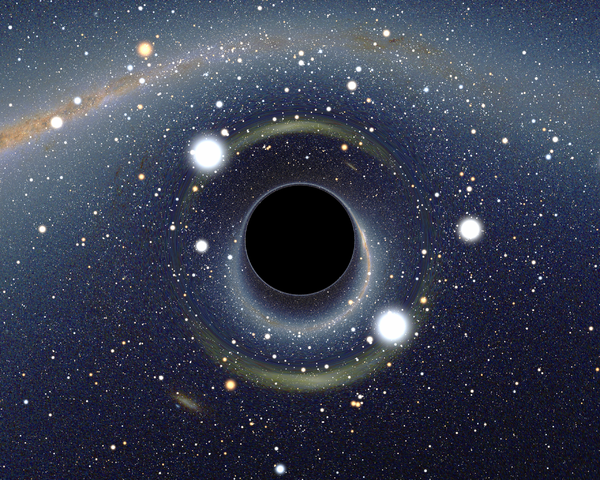# Earth = Black hole?If the Earth was to shrink down so as to form a black hole, what would be the black hole's (approximate) radius?

Details and Assumptions:

• $M_{e} = 6 \times 10^{24} \text{ kg}$.

• $G = 6.67 \times 10^{-11} \text{ N m}^{2}\text{ kg}^{-2}$.

• $c = 3 \times 10^{8} \text{ ms}^{-1}$.

×